#### Stata Webinar

Ready. Set. Go Stata. - June 13, 2023

## Tables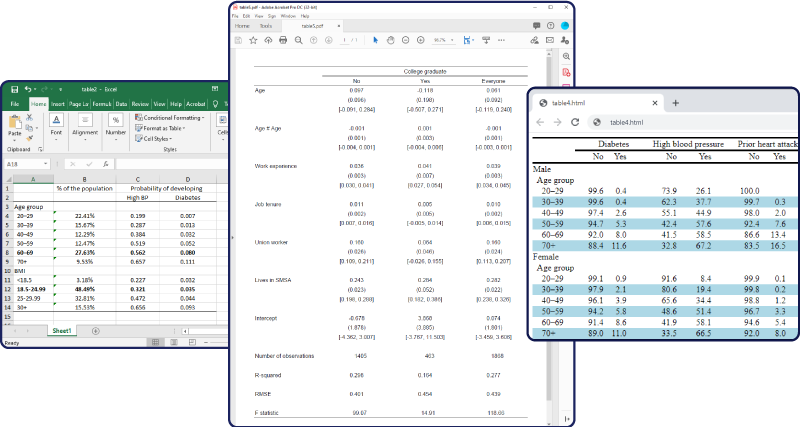• Summary statistics
• Results from hypothesis tests
• Regression results
• LR and Wald tests, GOF statistics, …
• Results from any Stata command

Export to

• Word, Excel
• LaTeX
• HTML, Markdown
• PDF
• and more

## Bayesian econometrics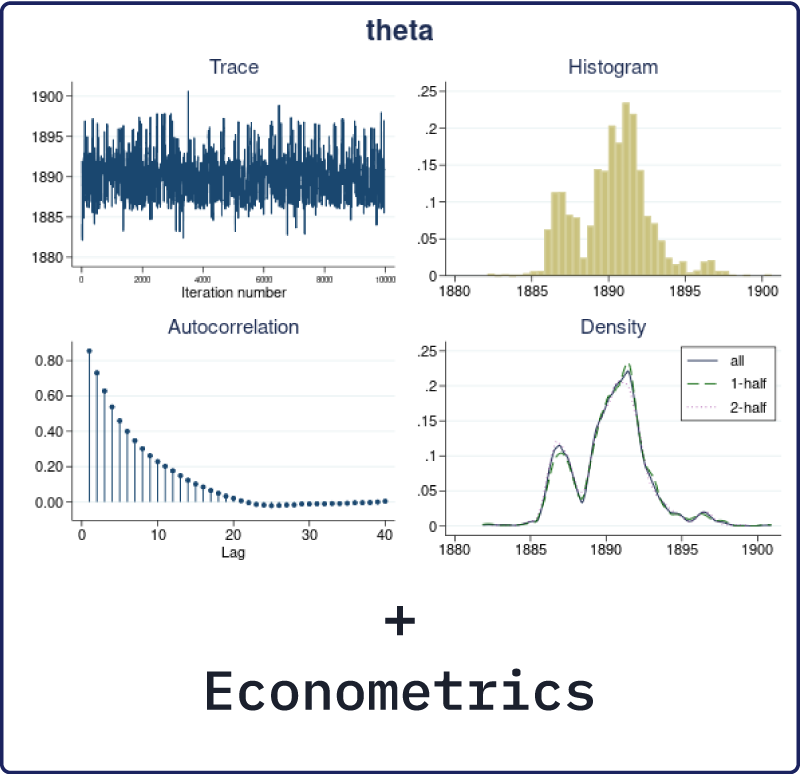Bayesian

• VAR models
• IRF and FEVD analysis
• Dynamic forecasting
• Panel/longitudinal-data models
• Linear and nonlinear DSGE models

## PyStata—Python and Stata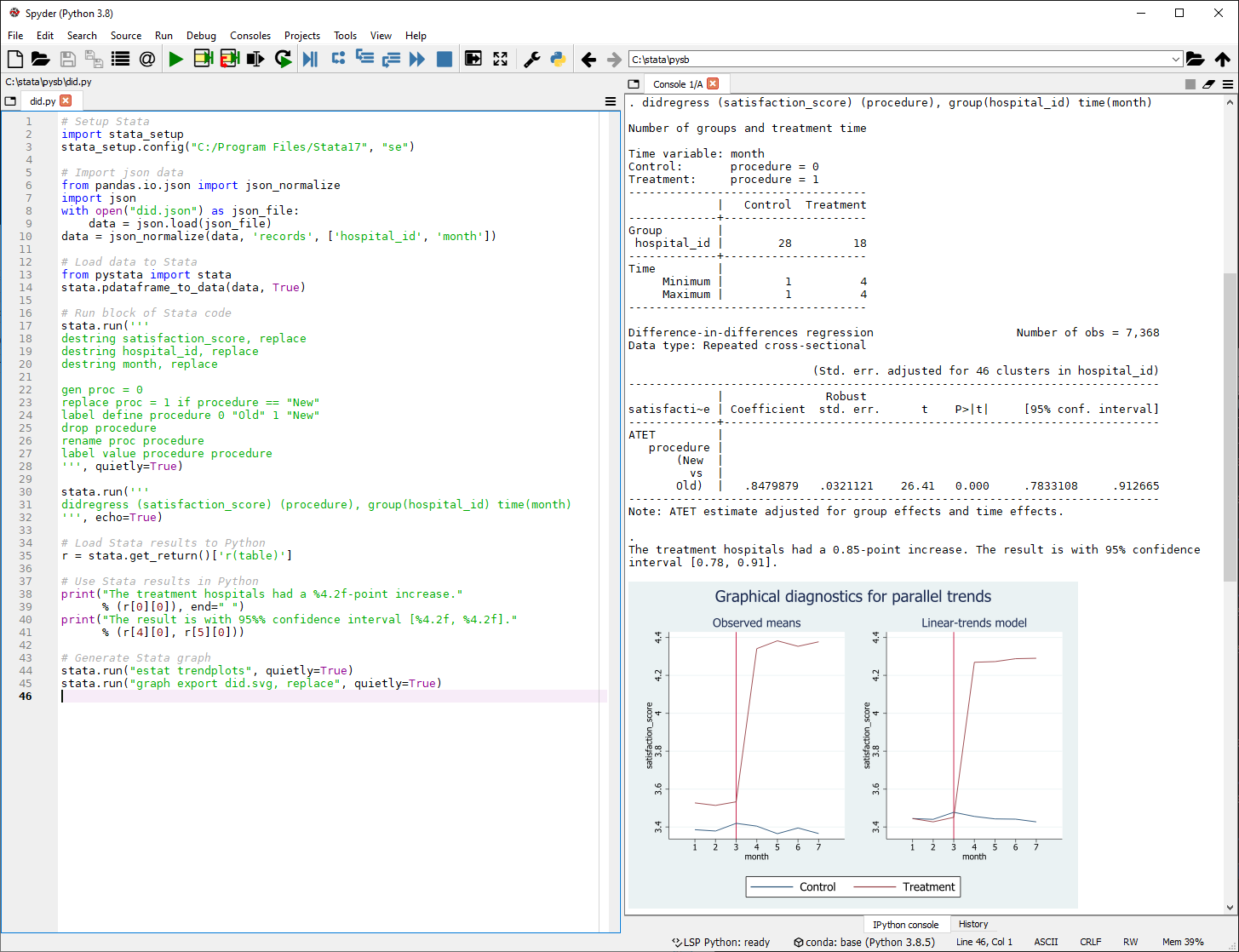• Call Python from Stata.
• Call Stata from Python.
• Exchange data, metadata, and results seamlessly.
• Use Stata from Jupyter Notebook, Spyder, PyCharm IDE, and more.

## Jupyter Notebook with Stata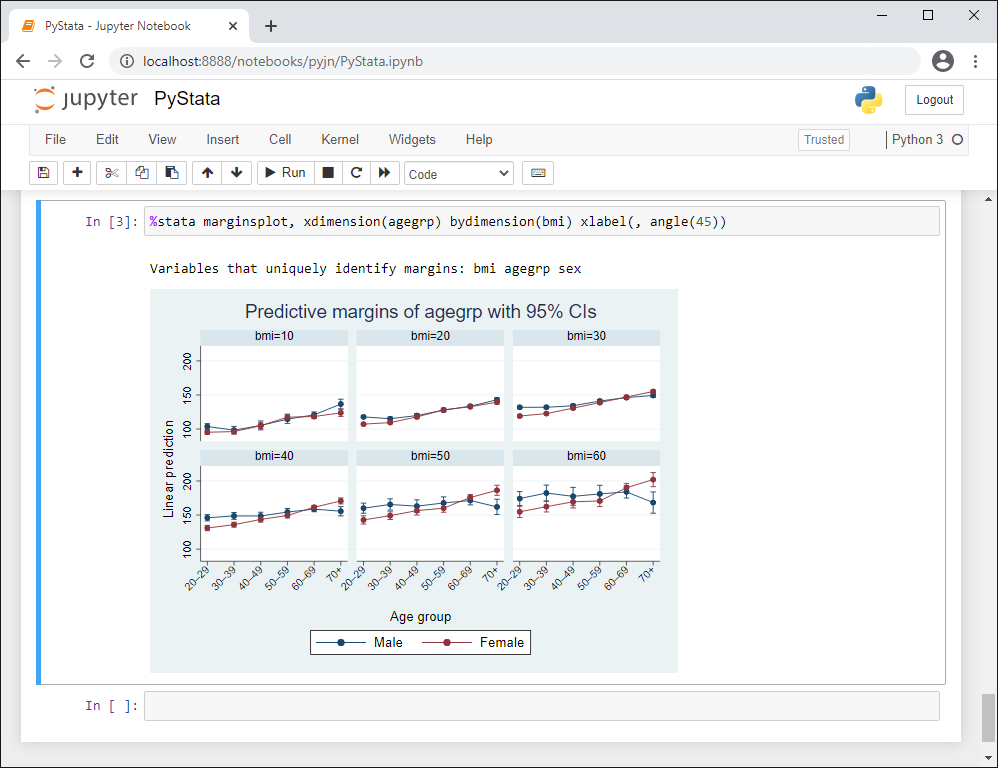• Invoke Stata and Mata from Jupyter Notebook.
• Easily reproduce your work and collaborate with others.
• Access results from Stata analyses within Python.
• Stata output, graphs, and tables seamlessly integrate with your Jupyter Notebook.

## Difference-in-differences (DID) and DDD models• Evaluate the effect of a policy, a treatment, or an intervention.
• Control for confounding unobserved group and time characteristics.
• Use panel data or repeated cross-sections.
• Use DID. In vogue since 1855.

## Faster Stata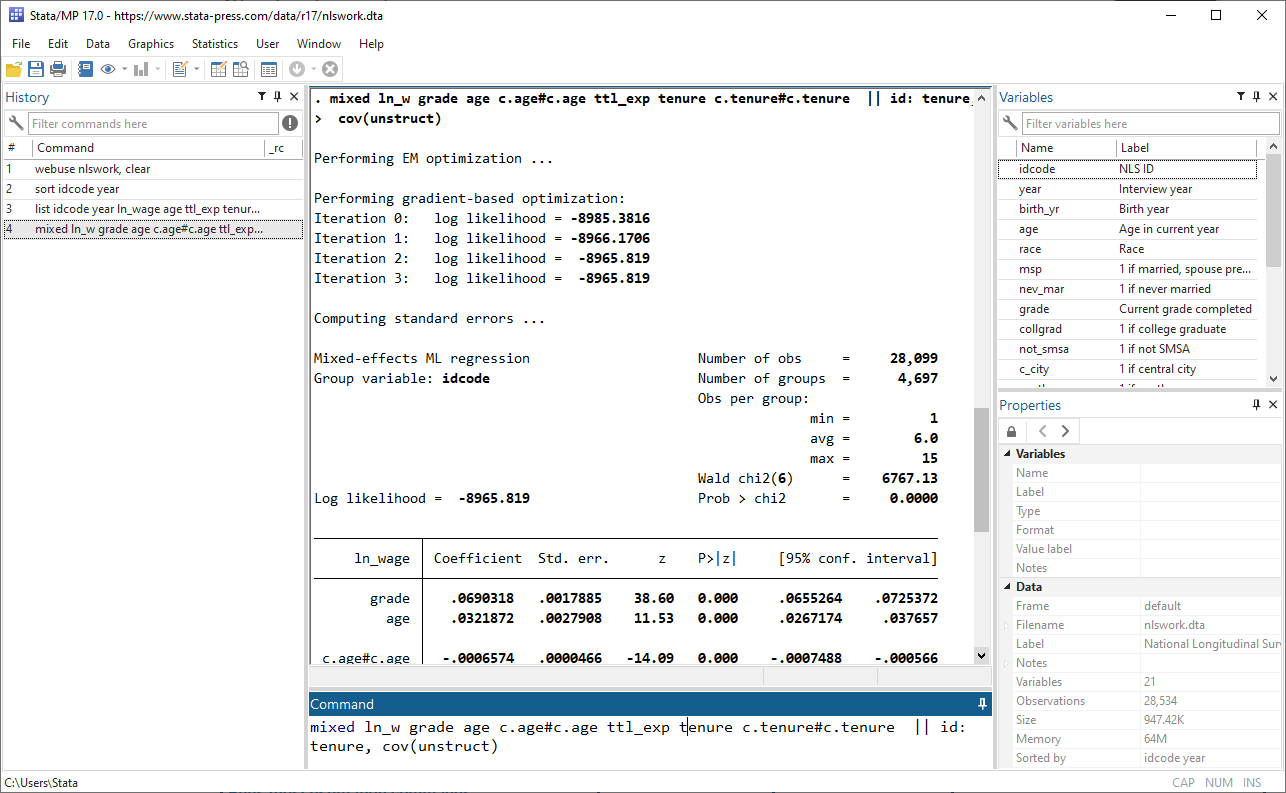Stata is fast, and keeps getting faster.

• Faster sort and collapse
• Faster mixed models
• Faster estimation commands
• Faster import delimited
• And more

## Interval-censored Cox modelYou want to model time to an event.

But you don’t know the exact event times—only the intervals in which events happen.

And you don’t want to make parametric assumptions.

Try an interval-censored Cox model.

## Multivariate meta-analysis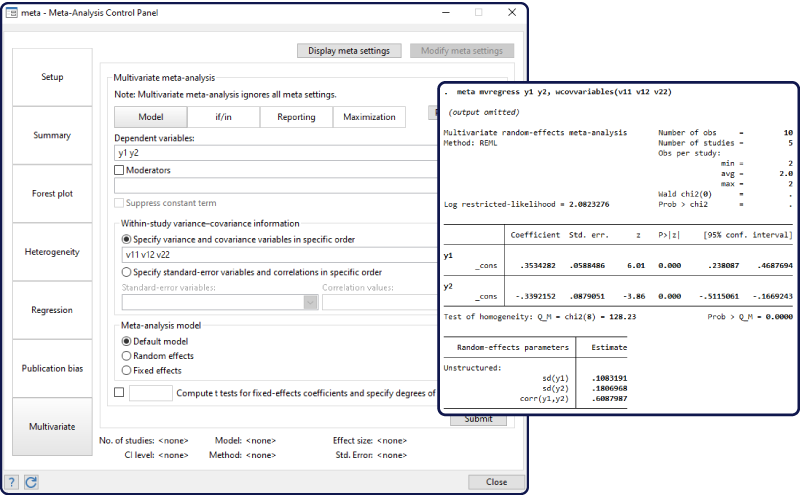Do you have multiple effect sizes?

Do they share a common control group?

Do they share the same group of subjects?

Multivariate meta-analysis can help.

## Bayesian VAR models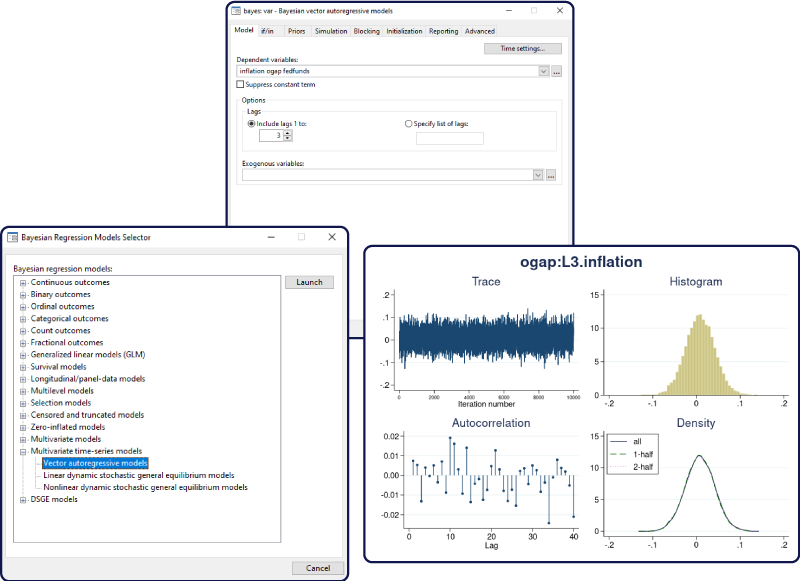You fit your VAR models with var.

You fit your Bayesian regression models with bayes:.

Now fit your Bayesian VAR models with bayes: var.

## Bayesian multilevel modelingNonlinear, joint, SEM-like, and more.

More multilevel models.

More powerful.

Easier to use.

## Treatment-effects lasso estimation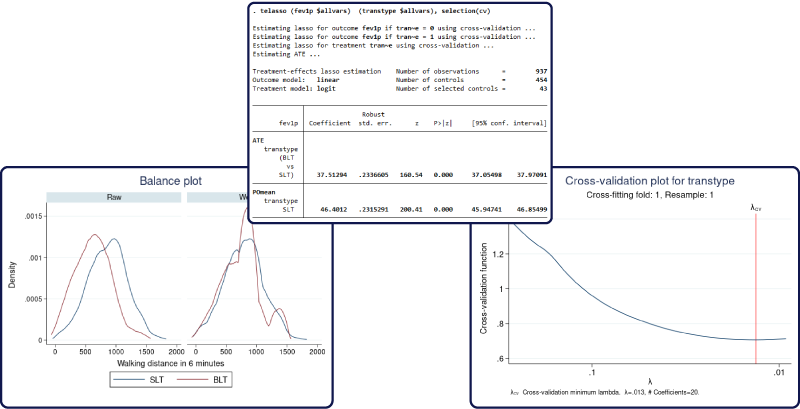When you want:

Causal inference, average treatment effects, potential-outcome means, double-robust estimation

And you have:

Many (maybe hundreds or thousands of) potential covariates

Use treatment-effects estimation with lasso variable selection.

## New functions for dates and times• Calculate durations, such as ages and other differences between datetimes.
• Calculate relative dates, or dates from other dates, such as the previous or next birthday or anniversary relative to a reference date.
• Extract individual components from datetime values and variables.

## Leave-one-out meta-analysis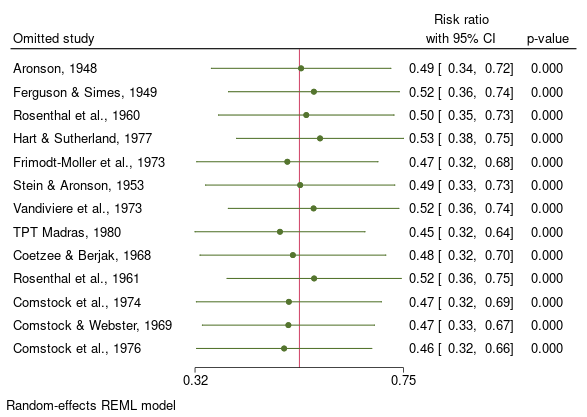Are there influential studies in your data?

Use leave-one-out meta-analysis to find out.

## Galbraith plotsGraphically summarize meta-analysis results

• Study-specific effect sizes
• Precision of effect sizes
• Overall effect size

Detect potential outliers

Assess heterogeneity

## Panel-data multinomial logit model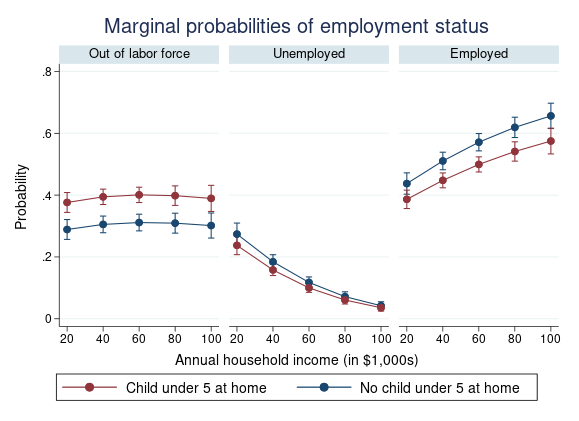You can model categorical outcomes with mlogit.

You can model panel data with xt.

Now you can do both!

Stata’s new xtmlogit command models categorical outcomes that change over time.

## Bayesian panel-data models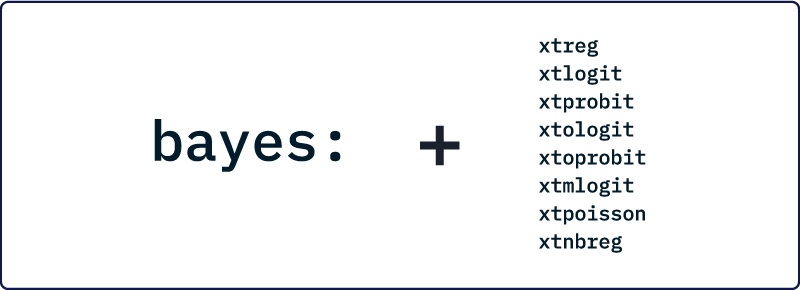Bayesian analysis lets you answer probabilistic questions with panel-data models.

• How likely is it that an extra year of schooling will increase wages?
• What is the probability of default for a low-risk portfolio?

Incorporate prior knowledge, see posterior distributions of random effects, compute Bayesian predictions, and more.

## Zero-inflated ordered logit modelNeed to model an ordinal outcome?

Have excess zeros (or responses in the lowest category)?

## Nonparametric tests for trend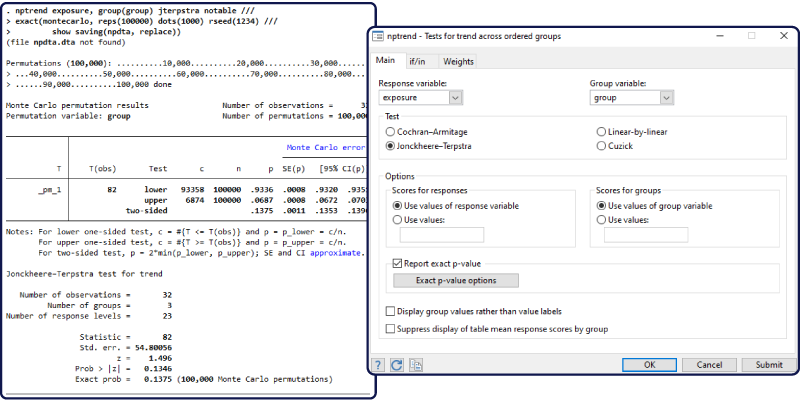Do responses have an increasing or decreasing trend? Find out using one of four nonparametric tests for trend:

• Cochran–Armitage test
• Jonckheere–Terpstra test
• Linear-by-linear test
• Cuzick’s test with ranks

## Bayesian IRF and FEVD analysis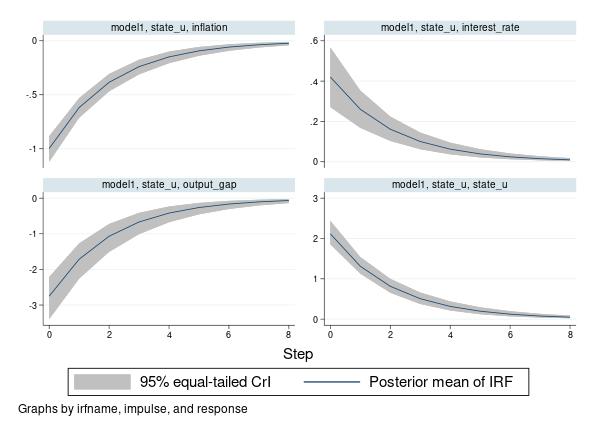What is the effect of a shock over time?

What is the mean or median of the effect for a distribution of probable scenarios?

Bayesian IRF analysis answers these and more.

## Bayesian dynamic forecasting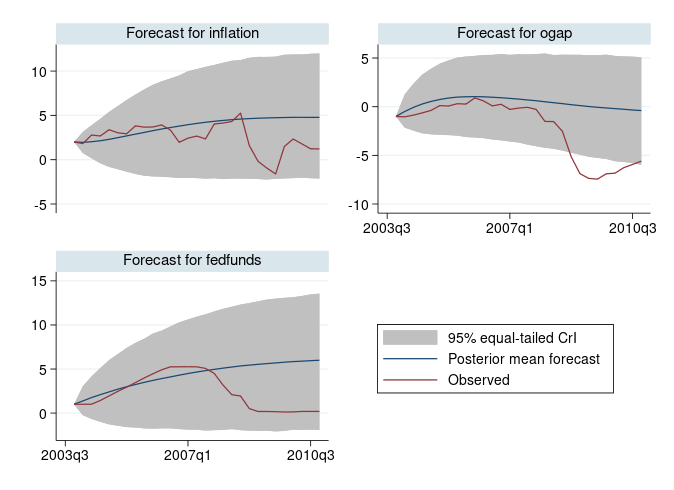After VAR, you want a dynamic forecast.

After Bayesian estimation, you want statistics of posterior distributions.

Estimate both. Visualize both.

## Lasso with clustered data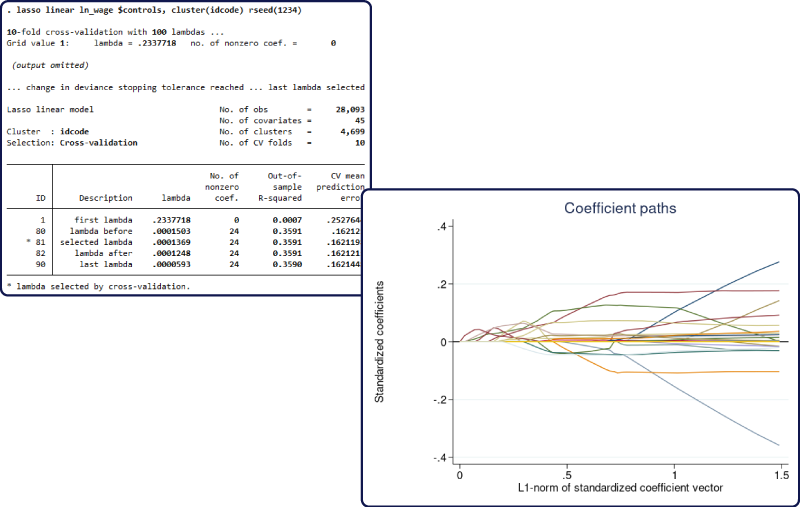many variables.

clusters of observations.

Your lasso for prediction, model selection, or inference can now select variables while accounting for clustering.

## BIC for lasso penalty selectionWhich variables should lasso include?

BIC for lasso penalty selection can tell you.

## Bayesian linear and nonlinear DSGE models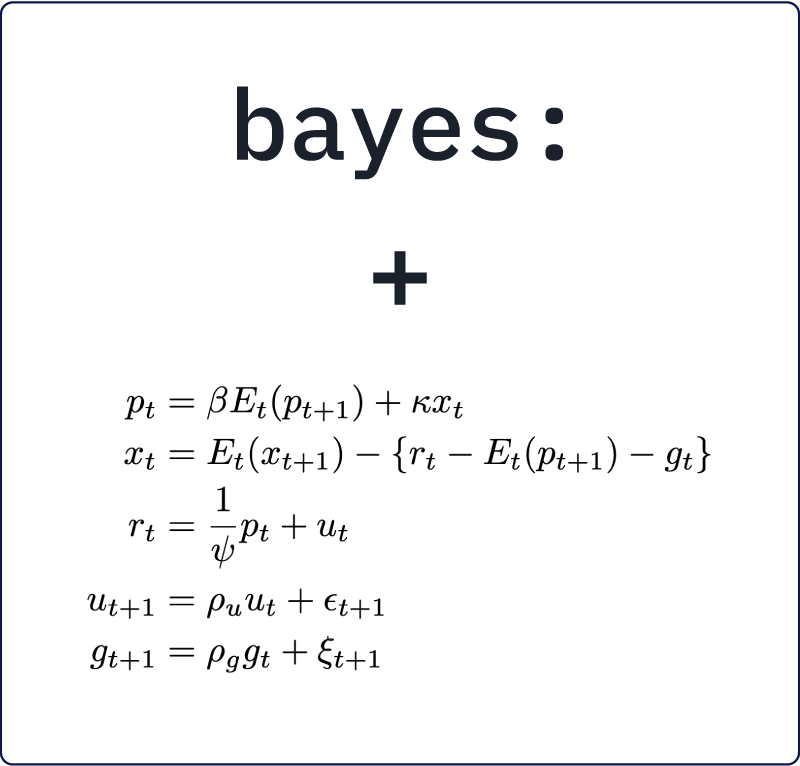Forming rational expectations
of the future is hard.

DSGE models include
these expectations.

Prior information helps.

## Do-file Editor enhancements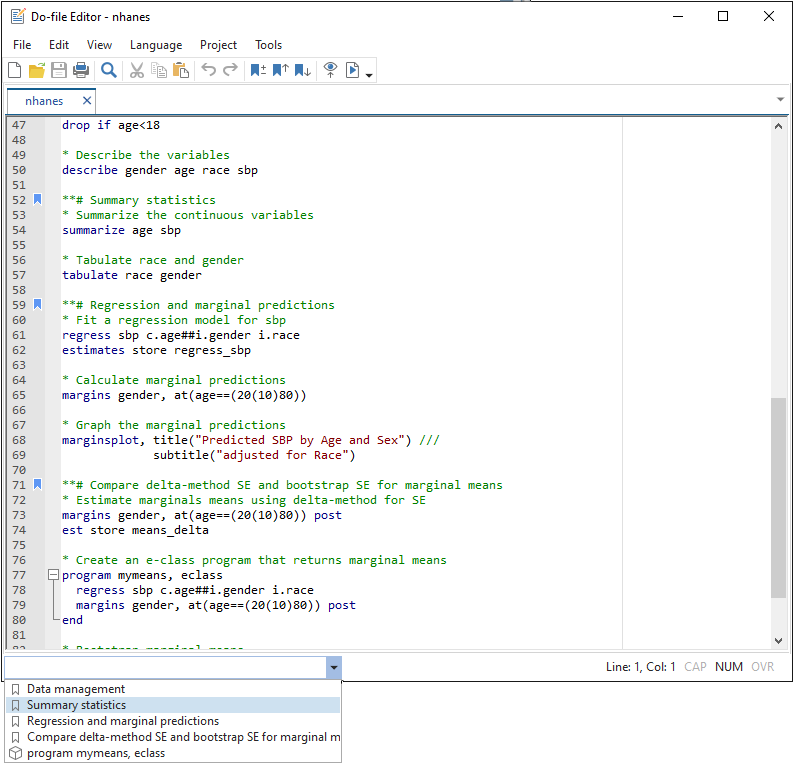• Persistent bookmarks
• Syntax highlighting for Java, XML, and more
• Auto-completion for quotes, parentheses, and brackets

## Stata on Apple Silicon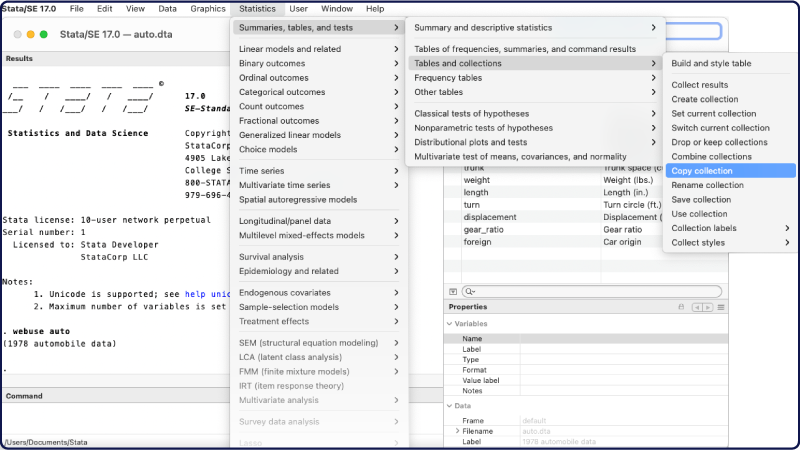• Native M1 processor support
• Universal application for both Intel and Apple Silicon Macs
• One license, both kinds of hardware

## Intel Math Kernel Library (MKL)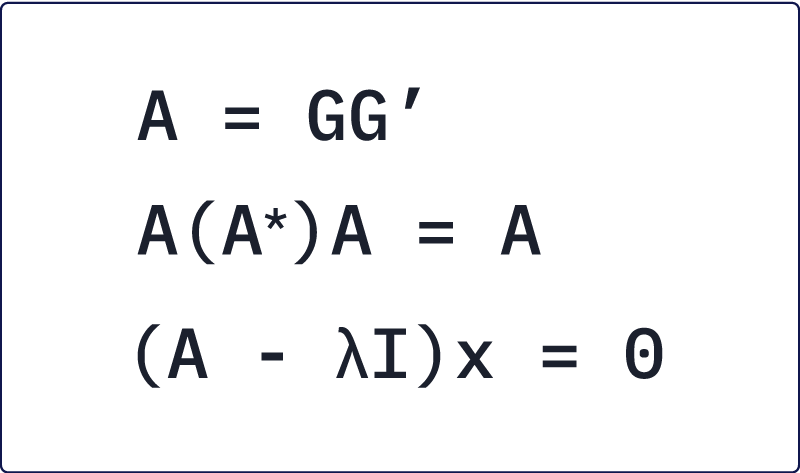Mata functions and operators use heavily optimized LAPACK routines underpinned by the Intel Math Kernel Library.

Use your favorite Stata commands like always; underlying functions are faster, so you get results faster.

## Java integration

• Use Java interactively (like JShell) from within Stata.
• Embed Java code in do-files.
• Embed Java code in ado-files.
• Compile and execute Java code “on the fly” without external programs.

## H2O integration

• Start a new H2O cluster or connect to an existing one.
• Manipulate data on an H2O cluster.
• Access the capabilities of H2O directly in Stata.

## JDBC

Connecting Stata to databases is now easier.

Want to access data from Oracle, MySQL, Amazon Redshift, Snowflake, Microsoft SQL Server, and others?

Use jdbc.

Want one driver that works on Windows, Mac, and Linux?

Use jdbc.